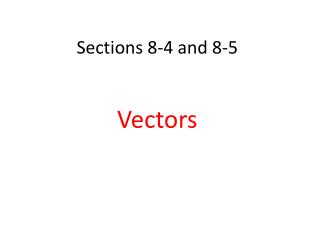DownloadDownload PresentationSections 8-4 and 8-5

# Sections 8-4 and 8-5

Download Presentation## Sections 8-4 and 8-5

- - - - - - - - - - - - - - - - - - - - - - - - - - - E N D - - - - - - - - - - - - - - - - - - - - - - - - - - -
##### Presentation Transcript

1. Sections 8-4 and 8-5 Vectors

2. Vocabulary • 1. Vector – a quantity that has both magnitude and direction. • 2. Magnitude – the length of the line segment. • 3. Direction – the directed angle between the positive x axis and the vector.

3. 4. Resultant – the sum of two or more vectors 5. Unit vector – a vector with magnitude of one unit • Inner product of vectors in a plane- 7. Inner product of vectors in space -

4. 8. Perpendicular vectors – vectors with an inner product of zero. • 9. Parallel vectors – vectors with the same direction and different magnitude • 10. Standard position – vectors with an initial point at the origin

5. Inner Product

6. (10,4)

7. 2, not perpendicular 13, not perpendicular 5, not perpendicular

8. Geometric VectorsSum of two vectors Parallelogram Method

9. Geometric VectorsSum of two vectorsTriangle Method

10. Triangle Method

11. One clown is exerting a force of 270 and the other is 360. (Use the parallelogram method.) Clown 1 Bull Clown 2

12. Determine the resultant forces.

13. Example 1, page 514

14. Lenny Montana was a former 5-year world heavy weight wrestling champion and actor. Suppose that Lenny and a tag team wrestling partner are each pulling horizontally and at a rt angle to each other on the arms of a wrestling opponent. Lenny exerts a force of 180 pounds due north while his partner exerts a force of 125 pounds due east.

15. Draw a labeled diagram that represents the forces.

16. Determine the resultant force exerted on the opponent. • 219 lb • Determine the angle the resultant force makes with the east-west axis. • 55.2° north of east

17. Textbook page 509 # 11-19 and • Textbook page 517 # 4,5,6 and #10-#15